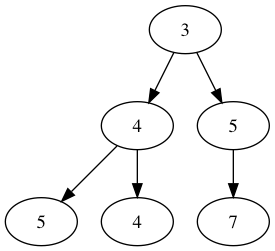# Introduction to Graphviz in Jupyter Notebook

## Goal¶

The goal in this post is to introduce graphviz to draw the graph when we explain graph-related algorithm e.g., tree, binary search etc. It would be nicer to have such a visualization to quickly digest problems and solutions.

Since we work with TreeNode and trees in a list-expresion e.g., [1, 2, null, 3] in LeetCode, the goal of this post is to easily convert the given tree in a list-expression into the visualization like below.

In :
from IPython.display import Image
Image('digraph.png')

Out: